﻿ Math geometry background stock image. Image of calculation - 12269465

# Math geometry background. Calculation, macro.

## Math geometry background with formulas, math, number equation

Designers also selected these stock photos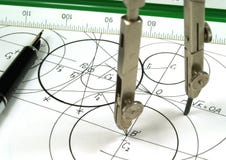GeometryTrapped ChildDoctor with microscope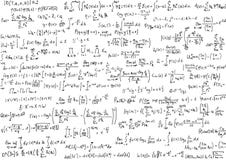Math equations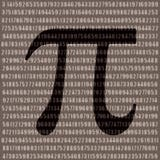Pi numberDoctor consults a young coupleBusiness person thinking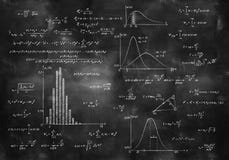Math physics formulas on chalkboardSemen test
More similar stock imagesSix degrees symbol in magnetic ball bearings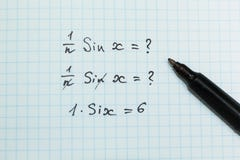Wrong solution of a mathematical example, math problemsScience word conceptSmile face with the yellow lightTransparent ruler with numbers on a white background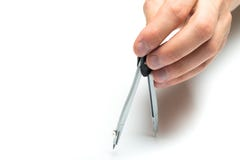Men`s hand holding architect compass on backgroung, close up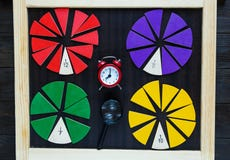Colorful math fractions on wooden background or table. interesting math for kids. Education, back to school concept. Geometry and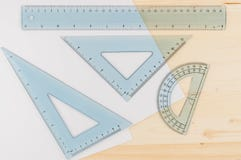Ruler Combo Set and Paper. Ruler, Protractor, Triangle, Top View, Close Up. Concept, Copy Space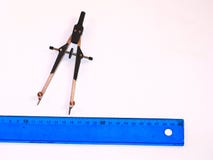Compasses and ruler on white background
More stock photos from Fernando Batista's portfolio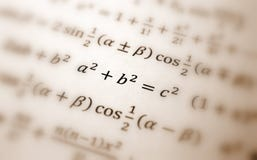Pythagoras equation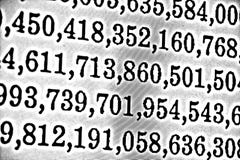White background HDR with many numbers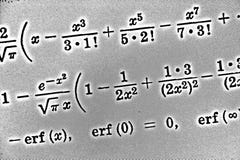Large number of mathematical formulas on white background HDR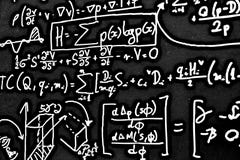Large number of mathematical formulas on white background HDR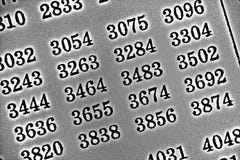White background HDR with many numbers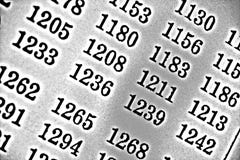White background HDR with many numbers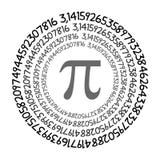The Pi symbol mathematical constant irrational number on circle, greek letter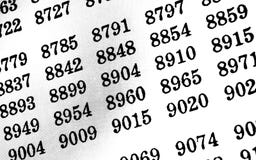White background with many numbers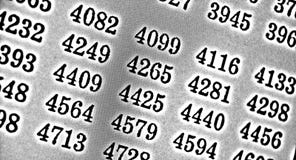White background HDR with many numbers
Related categories